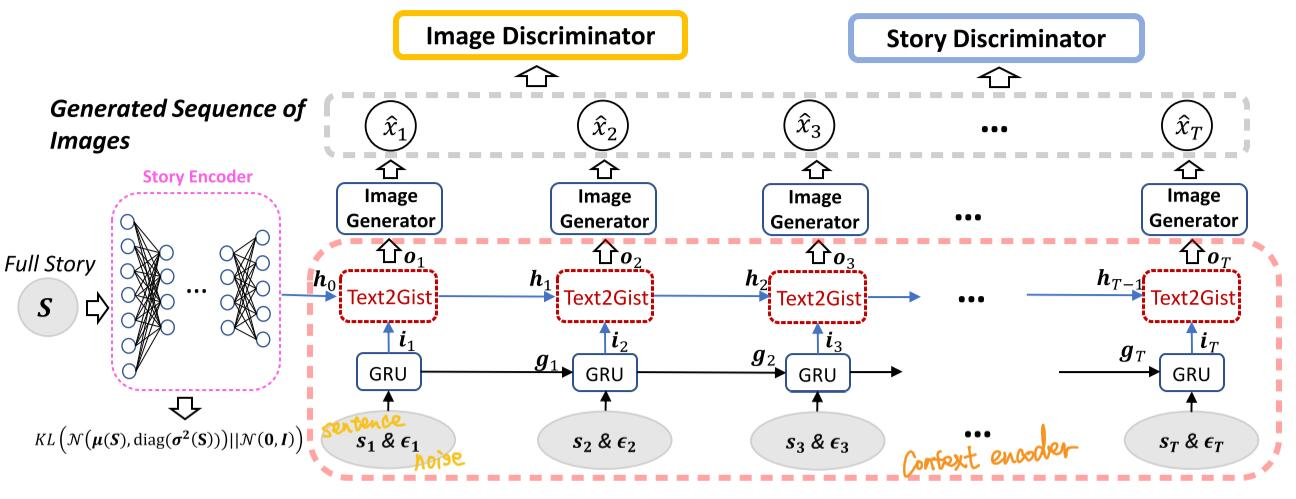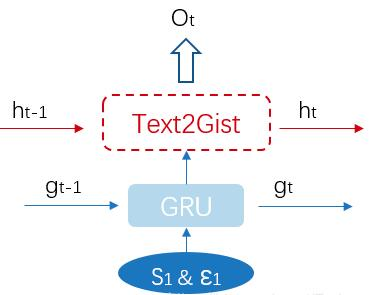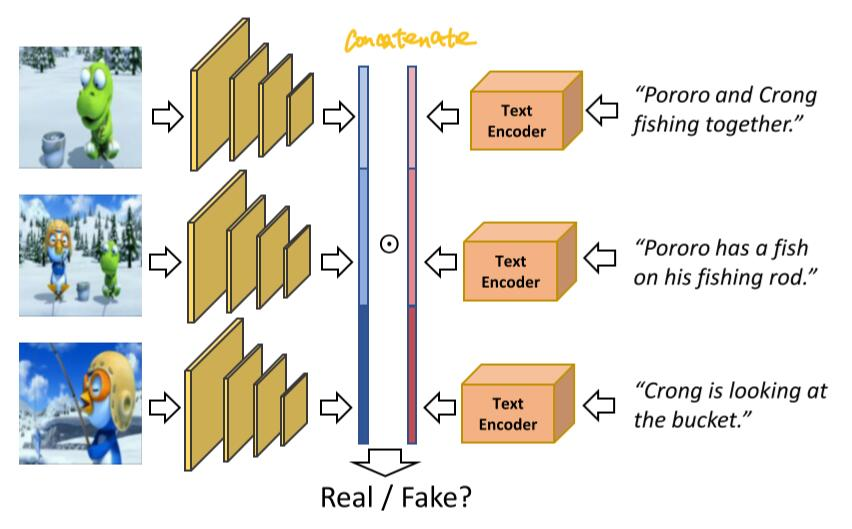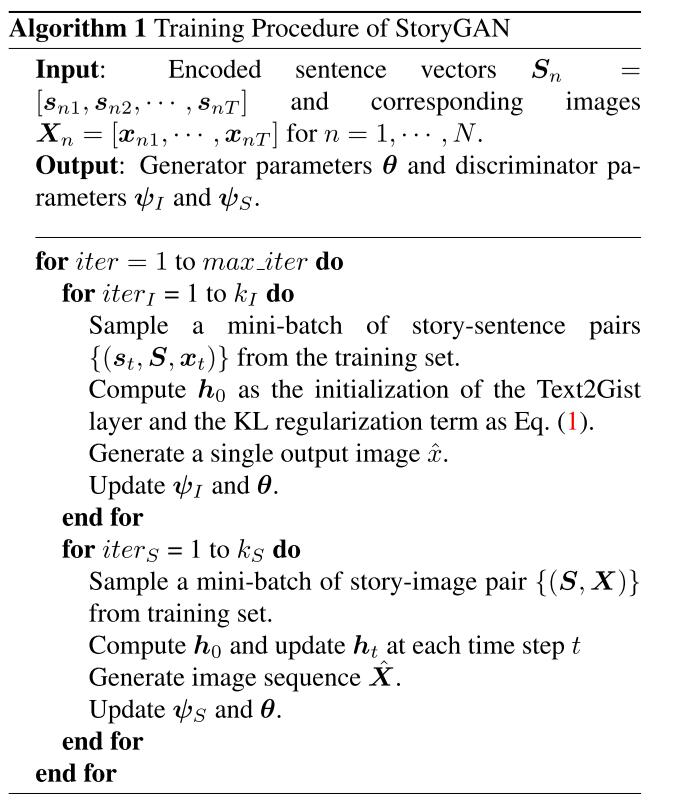# StoryGAN: A Sequential Conditional GAN for Story Visualization

## Overview

Given a paragraph consisting of multiple sentences, this paper proposed a sequential conditional GAN framework to generate a sequence of images, one for each sentence, to depict the total story.

Different from the video generation, story visualization mainly focuses less on the continuity of motions in frames, such as a running man move dynamically which is smoothly represented in the clip, but more on global consistency across scenes and characters.

## Main challenge

There are two main challenges for story visualization:

One is that the sequence of images should be coherently describe the total story. In other words, if the model is highly based on the previous strategy where each generation is solely based on the single sentence, the final result will be inconsistent. For instance, ‘A rectangular is at the center. Then add a round at the right’. In this case, the result will fail to represent the relationship between two object.

Another challenge is how to keep the appearance and layout of objects and background in a coherent way as the story progresses. For example, Harry and the surrounding around him appeared in the paragraph description should be uniform in some way.

## Difference between video generation and story visualization

For video generation, models focus on extract dynamic feature to maintain motions, like the man is running. It pay attention to the body movement as time goes by, while story visualization is devoted to a sequence of key static frames representing the whole plots, like this man runs from the garden with many flowers to the bus station and inquiry the people around him. From this point of view, motion features of story visualization are less important while scene changes with variation of multiple objects should be captured, comparing to the relatively static background in video clips.

## Architecture

The architecture is relatively easy to understand, as shown below. It is composed of Story Encoder, Context Enocder, Image Generator, Image Discriminator and Story Discriminator.### Story encoder

Story encoder is to map the story description into the low dimensional vector, story context $h_{0}$, which contains all information of the story.

Specifically, … TODO

To smooth the representation manifold in latent semantic space, KL divergence is imported.

$\mathcal{L}_{K L}=K L\left(\mathcal{N}\left(\boldsymbol{\mu}(\boldsymbol{S}), \operatorname{diag}\left(\boldsymbol{\sigma}^{2}(\boldsymbol{S})\right)\right) \| \mathcal{N}(\mathbf{0}, \boldsymbol{I})\right)$

where $S$ is the encoded feature vecotrs for the paragraph, different with the notation in the image for the convenience of interpretation.

### Context encoder

A deep RNN based Context encoder is imported to capture contextual information during sequential generation. It contains two parts, standard GRU cells and Text2Gist cells.For each time $t$, GRU takes as input sentence $s_{t}$ and noise $\epsilon_{t}$, and outputs $i_{t}$ which contains the local information of each sentence.

Then Text2Gist module combines $i_{t}$ with the story context $h_{t}$ to generate so-called ‘Gist’ vector $o_{t}$ which contains both global and local context information.

\begin{aligned} \boldsymbol{i}_{t}, \boldsymbol{g}_{t} &=\operatorname{GRU}\left(\boldsymbol{s}_{t}, \boldsymbol{\epsilon}_{t}, \boldsymbol{g}_{t-1}\right) \\ \boldsymbol{o}_{t}, \boldsymbol{h}_{t} &=\operatorname{Text} 2 \operatorname{Gist}\left(\boldsymbol{i}_{t}, \boldsymbol{h}_{t-1}\right) \end{aligned}

To be explicit for the Text2Gist, as shown below:

\begin{aligned} \boldsymbol{z}_{t} &=\sigma_{z}\left(\boldsymbol{W}_{z} \boldsymbol{i}_{t}+\boldsymbol{U}_{t} \boldsymbol{h}_{t-1}+\boldsymbol{b}_{z}\right) \\ \boldsymbol{r}_{t} &=\sigma_{r}\left(\boldsymbol{W}_{r} \boldsymbol{i}_{t}+\boldsymbol{U}_{r} \boldsymbol{h}_{t-1}+\boldsymbol{b}_{r}\right) \\ \boldsymbol{h}_{t} &=\left(\mathbf{1}-\boldsymbol{z}_{t}\right) \odot \boldsymbol{h}_{t-1} +\boldsymbol{z}_{t} \odot \sigma_{h}\left(\boldsymbol{W}_{h} \boldsymbol{i}_{t}+\boldsymbol{U}_{h}\left(\boldsymbol{r}_{t} \odot \boldsymbol{h}_{t-1}\right)+\boldsymbol{b}_{h}\right) \\ \boldsymbol{o}_{t} &=\text { Filter }\left(\boldsymbol{i}_{t}\right) * \boldsymbol{h}_{t} \end{aligned}

$z_{t}$ and $r_{t}$ are the update and reset gates, respectively. The update gate decide how much information from the previous step should be kept, while the reset gate determines what to forget from $h_{t-1}$.

$Filter\left(\boldsymbol{i}_{t}\right)$ is used to choose the necessary part for image generation. TODO

### Discriminator

Image and Story discriminator is introduced to ensure the local and global consistency of the story, respectively.

Image discriminator measures whether the generated image $\hat{\boldsymbol{x}}_{t}$ matches the sentecne $s_{t}$ by comparing triplet $\left\{s_{t}, h_{0}, \hat{x}_{t}\right\}$ to the real $\left\{s_{t}, h_{0}, x_{t}\right\}$

Story discriminator enforce the global consistency of image sequence given story $S$. It differs from video generation using 3D convolution to smooth the changes between frames. The framework is shown as follows:Each side of image or text descriptions are firstly embedded and concatenated separately in to two single vectors, shown as the blue and red one.

$D_{S}=\sigma\left(\boldsymbol{w}^{\top}\left(E_{i m g}(\boldsymbol{X}) \odot E_{t x t}(\boldsymbol{S})\right)+b\right)$

where $\odot$ is element-wise product.

## Training strategy

The objective for StoryGAN is:

$\min _{\boldsymbol{\theta}} \max _{\boldsymbol{\psi}_{I}, \boldsymbol{\psi}_{S}} \alpha \mathcal{L}_{\text {Image}}+\beta \mathcal{L}_{\text {Story}}+\mathcal{L}_{K L}$ \begin{aligned} \mathcal{L}_{\text {Image}} &=\sum_{t=1}^{T}\left(\mathbb{E}_{\left(\boldsymbol{x}_{t}, \boldsymbol{s}_{t}\right)}\left[\log D_{I}\left(\boldsymbol{x}_{t}, \boldsymbol{s}_{t}, \boldsymbol{h}_{0} ; \boldsymbol{\psi}_{I}\right)\right]\right.+\mathbb{E}_{\left(\boldsymbol{\epsilon}_{t}, \boldsymbol{s}_{t}\right)}\left[\log \left(1-D_{I}\left(G\left(\boldsymbol{\epsilon}_{t}, \boldsymbol{s}_{t} ; \boldsymbol{\theta}\right), \boldsymbol{s}_{t}, \boldsymbol{h}_{0} ; \boldsymbol{\psi}_{I}\right)\right)\right] ) \\ \mathcal{L}_{S t o r y} &=\mathbb{E}_{(\boldsymbol{X}, \boldsymbol{S})}\left[\log D_{S}\left(\boldsymbol{X}, \boldsymbol{S} ; \boldsymbol{\psi}_{S}\right)\right]+\mathbb{E}_{(\epsilon, \boldsymbol{S})}\left[\log \left(1-D_{S}\left(\left[G\left(\boldsymbol{\epsilon}_{t}, \boldsymbol{s}_{t} ; \boldsymbol{\theta}\right)\right]_{t=1}^{T}, \boldsymbol{S} ; \boldsymbol{\psi}_{S}\right)\right)\right] \end{aligned}

where $\theta$, $\psi_{I}$ and $\psi_{S}$ denotes the parametes of generator, image discriminator and story discriminator, respectively.

The algorithm outlines is given: